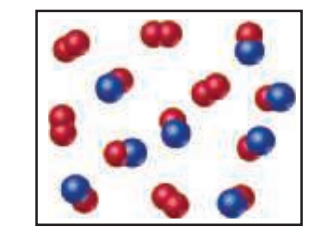# ?Nitrogen monoxide and oxygen react to form nitrogen dioxide. Consider the mixture of NO and $$\mathrm{O}_{2}$$ shown in the accompanying diagram. The

Chapter 3, Problem 3.8

(choose chapter or problem)

Nitrogen monoxide and oxygen react to form nitrogen dioxide. Consider the mixture of NO and $$\mathrm{O}_{2}$$ shown in the accompanying diagram. The blue spheres represent N, and the red ones represent O.

(a) How many molecules of $$\mathrm{NO}_{2}$$ can be formed, assuming the reaction goes to completion?

(b) What is the limiting reactant?

(c) If the actual yield of the reaction was 75% instead of 100%, how many molecules of each kind would be present after the reaction was over?Text Transcription:

O_2

NO_2

Unfortunately, we don't have that question answered yet. But you can get it answered in just 5 hours by Logging in or Becoming a subscriber.